• 该程序生成一个二维高斯。 然后程序尝试使用 MatLab 函数“lsqcurvefit”拟合数据，以找到二维高斯的位置、方向和宽度。... 二维高斯函数由函数“D2GaussFunctionRot.m”和“D2GaussFunction.m”定义matlab
• ## 二维高斯函数

千次阅读 2018-08-25 12:28:34
https://blog.csdn.net/farmwang/article/details/78699926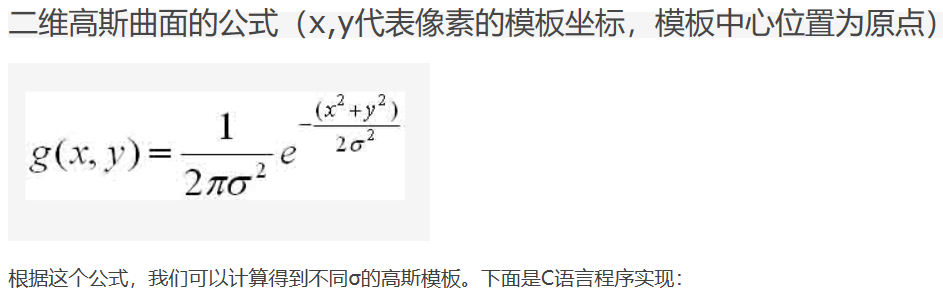https://blog.csdn.net/farmwang/article/details/78699926
展开全文• 这篇文章以通俗易懂的语言和方式解释了如何...2、根据一维高斯函数，可以推导得到二维高斯函数：   在图形上，正态分布是一种钟形曲线，越接近中心，取值越大，越远离中心，取值越小。 计算平均值的时

这篇文章以通俗易懂的语言和方式解释了如何使用高斯函数模糊一张图片。

1、一维高斯函数：

a表示得到曲线的高度，b是指曲线在x轴的中心，c指width(与半峰全宽有关),图形如下：2、根据一维高斯函数，可以推导得到二维高斯函数：在图形上，正态分布是一种钟形曲线，越接近中心，取值越大，越远离中心，取值越小。
计算平均值的时候，我们只需要将"中心点"作为原点，其他点按照其在正态曲线上的位置，分配权重，就可以得到一个加权平均值。例如：通常，图像处理软件会提供"模糊"（blur）滤镜，使图片产生模糊的效果。"高斯模糊"（Gaussian Blur）。它将正态分布（又名"高斯分布"）用于图像处理。数据平滑技术（data smoothing），适用于多个场合，图像处理恰好提供了一个直观的应用实例。

高斯模糊的原理

所谓"模糊"，可以理解成每一个像素都取周边像素的平均值。上图中，2是中间点，周边点都是1。"中间点"取"周围点"的平均值，就会变成1。在数值上，这是一种"平滑化"。在图形上，就相当于产生"模糊"效果，"中间点"失去细节。显然，计算平均值时，取值范围越大，"模糊效果"越强烈。上面分别是原图、模糊半径3像素、模糊半径10像素的效果。模糊半径越大，图像就越模糊。从数值角度看，就是数值越平滑。

接下来的问题就是，既然每个点都要取周边像素的平均值，那么应该如何分配权重呢？

如果使用简单平均，显然不是很合理，因为图像都是连续的，越靠近的点关系越密切，越远离的点关系越疏远。因此，加权平均更合理，距离越近的点权重越大，距离越远的点权重越小。

正态分布的权重

正态分布显然是一种可取的权重分配模式。在图形上，正态分布是一种钟形曲线，越接近中心，取值越大，越远离中心，取值越小。

计算平均值的时候，我们只需要将"中心点"作为原点，其他点按照其在正态曲线上的位置，分配权重，就可以得到一个加权平均值。

高斯函数

上面的正态分布是一维的，图像都是二维的，所以我们需要二维的正态分布。"高斯函数"（Gaussian function）。它的一维形式是其中，μ是x的均值，σ是x的方差。因为计算平均值的时候，中心点就是原点，所以μ等于0。根据一维高斯函数，可以推导得到二维高斯函数有了这个函数 ，就可以计算每个点的权重了。

权重矩阵

假定中心点的坐标是（0,0），那么距离它最近的8个点的坐标如下：更远的点以此类推。

为了计算权重矩阵，需要设定σ的值。假定σ=1.5，则模糊半径为1的权重矩阵如下：这9个点的权重总和等于0.4787147，如果只计算这9个点的加权平均，还必须让它们的权重之和等于1，因此上面9个值还要分别除以0.4787147，得到最终的权重矩阵。计算高斯模糊

有了权重矩阵，就可以计算高斯模糊的值了。

假设现有9个像素点，灰度值（0-255）如下：每个点乘以自己的权重值：得到将这9个值加起来，就是中心点的高斯模糊的值。

对所有点重复这个过程，就得到了高斯模糊后的图像。如果原图是彩色图片，可以对RGB三个通道分别做高斯模糊。

转载自：http://www.cnblogs.com/herenzhiming/articles/5276106.html

展开全文• 本篇博文记录使用MATLAB绘制二维高斯函数的三维图。 用到的MATLAB函数——mesh()（绘制三维线框图）和surf()（绘制三维表面图）。 MATLAB命令窗口输入&gt;&gt; doc 函数名，可以查看相关函数的帮助文档。...
本篇博文记录使用MATLAB绘制二维高斯函数的三维图。

用到的MATLAB函数——mesh()（绘制三维线框图）和surf()（绘制三维表面图）。

MATLAB命令窗口输入>> doc 函数名，可以查看相关函数的帮助文档。

一维高斯函数：

（μ为均值，σ为标准差）

二维高斯函数：

（这里，均值均为零，标准差均为σ）

下面给出代码（两种实现结果），绘制一个均值为25，标准差为5的二维高斯函数的三维图：

代码实现一：

% 先限定三维图中的x,y轴坐标范围
X = 0 : 1 : 50;
Y = 0 : 1 : 50;

% 标准差
sigma = 5;

Z = zeros( 51, 51 );
for row = 1 : 1 : 51
for col = 1 : 1 : 51
Z( row, col ) = ( X(row) - 25 ).^2 + ( Y(col) - 25 ).^2; % 均值(25, 25)
end
end
Z = -Z / ( 2 * sigma^2 );

Z = exp(Z) / ( 2 * pi * sigma^2 );

% 显示高斯函数的三维曲面
figure, surf(X, Y, Z);

结果图：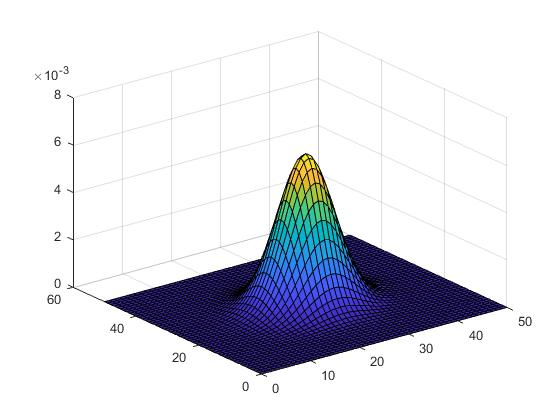代码实现二：（更推荐）

% 先限定三维图中的 x,y 轴坐标范围
X = 0 : 1 : 50;
Y = 0 : 1 : 50;

% 标准差
sigma = 5;

[ XX, YY ] = meshgrid( X, Y );
Z = ( XX - 25 ).^2 + ( YY - 25 ).^2;

Z = -Z / ( 2 * sigma^2 );
Z = exp(Z) / ( 2 * pi * sigma^2 );

% 显示高斯函数的三维图
figure, mesh(X, Y, Z); % 线框图

结果图：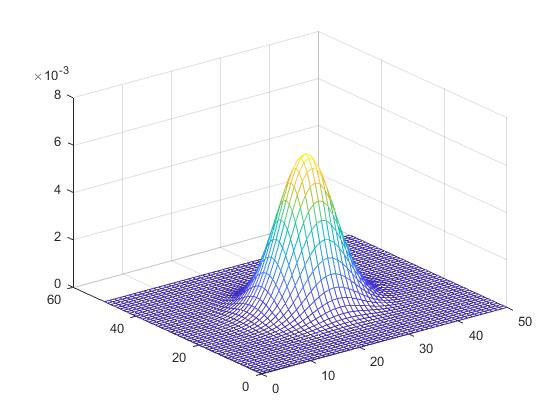以上两种代码实现中，差别在于Z的计算。代码实现一中，使用了双层for循环，时间会比代码二长。而代码实现二中，利用向量化运算取代for循环（即向量化循环），代码的效率会更高，更值得推荐。（这也是MATLAB编程上的一个技巧）。

并且，使用meshgrid，不会受到坐标（索引）为零的影响。例如：

% 先限定三维图中的 x,y 轴坐标范围
X = -25 : 1 : 25;
Y = -25 : 1 : 25;

% 标准差
sigma = 5;

[ XX, YY ] = meshgrid( X, Y );
Z = ( XX ).^2 + ( YY ).^2; % 均值为（0,0）

Z = -Z / ( 2 * sigma^2 );
Z = exp(Z) / ( 2 * pi * sigma^2 );

% 显示高斯函数的三维图
figure, mesh(X, Y, Z); % 线框图

结果图：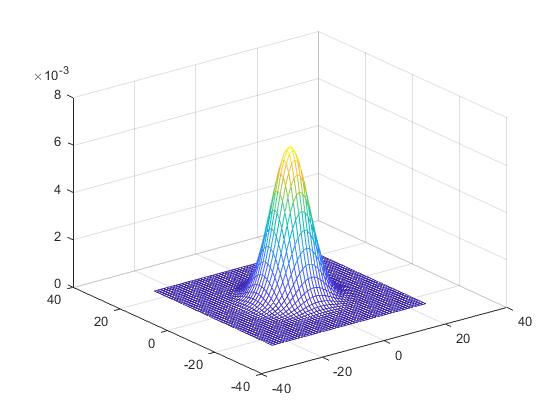以上三维图中，MATLAB默认绘制彩色图。

colormap( [0 0 0] ); % 或者 colormap( gray ) —— 可以将以上彩色三维图转换为灰色。

另外，还可控制三维图中的网格显示和坐标轴显示。

grid off; % 关闭网格 （grid on —— 开启网格）

axis off; % 关闭坐标轴显示 （axis on —— 开启坐标轴显示）

以上内容可用于二维滤波器的三维可视化。相关内容在书籍《数字图像处理（MATLAB版）》“4.5 在频域中直接生成滤波器”章节也有讲述。

下面的这段文字是对上述向量化循环的扩展，文字引自《数字图像处理（MATLAB版）》/（美）冈萨雷斯等著；阮秋琦等译.北京：电子工业出版社，2005,9.

MATLAB使用函数meshgrid来实现二维函数的评估，该函数的语法为：

[ C, R ] = meshgrid( c, r );

该函数将由行向量c和r指定的域变换为数组C和R，这两个数组能用来评估有两个变量的函数和三维表面图（注意，在meshgrid的输入输出中，列总是首先列出）。

输出数组C的行是向量c的副本，输出数组R的列是向量r的副本。

例如，假设我们想形成一个二维函数，该函数的元素是坐标变量x和y的值的平方和，其中，x=0,1,2  y=0,1。向量r由坐标的行分量构成：r=[0,1,2]；类似地，向量c由坐标的列分量构成：c=[0,1]，这里r和c均为行向量。

将这两个向量代入meshgrid，可得如下数组：

>>[ C, R ] = meshgrid( c, r )

C =

0     1

0     1

0     1

R =

0      0

1      1

2      2

>>h = R .^2 +C .^2

h =

0      1

1      2

4      5

h的维度为length(r) * length(c)

参考：

 Matlab绘制三维曲面（以二维高斯函数为例）（https://www.cnblogs.com/pzxbc/archive/2012/02/14/2351708.html）

在进行以上内容的学习时，也检索到下面的写得不错的博客：

（1）高斯函数以及在图像处理中的应用总结（https://www.cnblogs.com/herenzhiming/articles/5276106.html）

（2）高斯函数的详细分析（https://blog.csdn.net/jorg_zhao/article/details/52687448）


展开全文• 二维高斯函数数学公式如下： （u1是原点x坐标） （u2是原点y坐标） 是各个点所占的权重，在图像处理中，一般u1,u2是远点坐标。 例如当sigma=1时，可得权重去下： [0.36787945, 0.60653067, 0.36787945; 0...
二维高斯函数数学公式如下：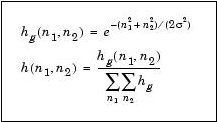（u1是原点x坐标）

（u2是原点y坐标）

是各个点所占的权重，在图像处理中，一般u1,u2是远点坐标。

例如当sigma=1时，可得权重去下：

[0.36787945, 0.60653067, 0.36787945;
0.60653067, 1, 0.60653067;
0.36787945, 0.60653067, 0.36787945]

这里只是各个点所占权重，还需要将该权重映射到具体像素值上，这里只需要用对应坐标上像素与对应位置上的权重做乘积求和作为原点的当前像素值。

Cpp代码如下:

Mat Gass_Kernel(float sigma)
{
Mat Kernel = Mat(Size(3, 3), CV_32FC1);
float val_sum = 0.f;
for (int i=0;i<3;i++)
for (int j = 0; j < 3; j++)
{
float val = exp(-((i - 3/2)*(i - 3/2) + (j - 3/2)*(j - 3/2)) / (2 * sigma*sigma));
Kernel.at<float>(i, j) = val;
val_sum += val;
}
cout << Kernel << endl;
cout << val_sum << endl;

for (int i = 0; i<3; i++)
for (int j = 0; j < 3; j++)
{
Kernel.at<float>(i, j) = Kernel.at<float>(i, j)/ val_sum;
}
return Kernel;

}

用上述代码计算道德filter_kernel做卷积，提取图像特征。

Mat Conv(Mat &map, Mat & kernel)
{
int new_h =int((map.rows - kernel.rows)/1)+1;
int new_w= int((map.cols - kernel.cols) /1) + 1;
Mat new_mat = Mat(Size(new_h, new_w), map.depth());
for (int i = 1; i < map.rows-1; i++)
{
for (int j = 1; j < map.cols - 1; j++)
{

//卷积计算
float value = 0.f;
for (int k_i=-1 ;k_i<=1 ;k_i++)
for (int k_j = -1; k_j <= 1; k_j++)
{
value += kernel.at<uchar>(k_i + 1, k_j + 1)*map.at<uchar>(i + k_i, j + k_j);
}
new_mat.at<uchar>(i, j) = uchar(value);
}
}

return new_mat;
}


展开全文• 原文地址为：Matlab绘制三维曲面（以二维高斯函数为例） 　寒假学习了一下Python下的NumPy和pymatlab，感觉不是很容易上手。来学校之后，决定继续看完数字图像处理一书。还是想按照上学期的模式，边看边实现书中的...
• Matlab 的曲面拟合（还）不... 它还接受定义下限、上限以及 7 个拟合参数的最佳猜测的结构作为第个输入。 该结构有 3 个字段：“Lower”、“StartingPoint”和“Upper”。 标题字符串可以作为第三个输入参数提供。matlab
• 二维高斯函数表达式为： 程序如下： u=[-10:0.1:10]; v=[-10:0.1:10]; [U,V]=meshgrid(u,v); H=exp(-(U.^2+V.^2)./2/3^2); mesh(u,v,H); %绘制三维曲面的函数 title('高斯函数曲面'); 运行结果为：
• 二维高斯函数 %code fgs = @(x,y)exp(-(sqrt(x.^2+y.^2)-70).^2./(2*30.^2)); %圆环半径70F2 = fgs(xp,yp);imagesc(F2) 显示： 二维正弦条纹图： %code 三个相位 clc,clear[xp,yp] = meshgrid(-100:100,...
• 可建立原始图像的尺度空间模型 二维高斯函数 我们使用的是图像，因此我们考虑二维高斯函数 高斯模糊是一种图像滤波器，它使用高斯函数计算模糊模板，并使用该模板与原图像做卷积运算，达到模糊图像的目的。...SIFT 尺度空间 高斯金字塔 ai
• 用于计算二维高斯 Q 函数的 Matlab 代码。 一般说明这个 m 文件提供了二维高斯 Q 函数的数值计算，即Q(x1,x2,ro)=1/((1/sqrt(2*pi)*(1-ro^2))*int(int(exp(-(x^2+y^2-2roxy)/ (2*(1-ro^2))) dxdy 结果可能包括非常...matlab
•python opencv
• 目前利用水下地理信息对惯导误差进行校正多以分类匹配算法为核心，由此论文提出一种基于二维高斯样条函数的水下重力被动定位新模式。二维高斯样条函数逼近的连续局部重力场模型，随后在该模型的基础上将实测重力表示...
• 当提供均值和协方差时，此函数绘制二维多变量高斯图。 它不使用 for 循环。 例如：绘图平均值= [10; 11]，cov = [6 0; 0 6] 2D多元高斯函数>> mvg([10;11],[6 0;0 6])matlab
• ## 二维高斯核

千次阅读 2017-10-18 16:51:37
标准差为σ的二维高斯函数如下： G(x,y)=e^(-(x^2+y^2)/(2σ^2 ))  注意此公式和标准的高斯概率密度函数的定义相差一个常数项。 为确定高斯滤波器大小，采用位于二维高斯表面下其均值在±3σ之间的99.7%。这样...
• 1.3 二维高斯函数 2、高斯模糊原理 2.1 二维高斯函数求权重 2.2 权重矩阵 2.3 计算高斯模糊 3、高斯核函数 3.2径向基函数RBF 3.3 高斯函数性质 4、高斯噪声 4.1 噪声 4.2 高斯噪声 ...
• 二维高斯正态分布函数(原创) 二维高斯正态分布函数在很多地方都用的到，比如说在滤波中，自己编了个，但感觉IDL中应该有现成的函数？？（我没找到）。如有，请高手指点。 ;--------------------------...
• 函数 ret = drawBayesGauss2D(mu,c,prProb,ax) % 绘制二维高斯分布的贝叶斯分类结果。 % mu：2×N，N 个类别的平均向量。 % c：2×2×N，cov 矩阵。 % prProb：1×N，先验概率。 % ax: [xmin xmax ymin ymax]，绘制...matlab
• 二维高斯函数表达式为： 程序如下： clc; sig=1.5; w_x=(-5:0.1:5); w_y=(-5:0.1:5); [X,Y]=meshgrid(w_x,w_y); W=exp(-(X.^2+Y.^2)./sig.^2); mesh(w_x,w_y,W); %绘制三维曲面的函数图像处理 matlab
• 高斯函数以及在图像处理中的应用总结 1、一维高斯函数： a表示得到曲线的高度，b是指曲线在x轴的...2、根据一维高斯函数，可以推导得到二维高斯函数：   在图形上，正态分布是一种钟形曲线，越接近中心，...图像处理
• OpenCV二维高斯滤波核实现 matlab的fspecial函数，与matlab结果一致opencv matlab...